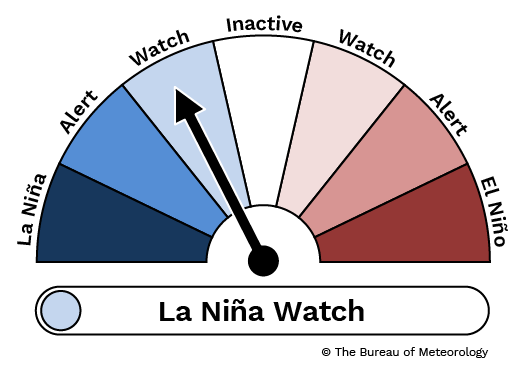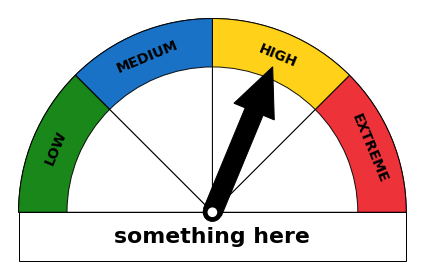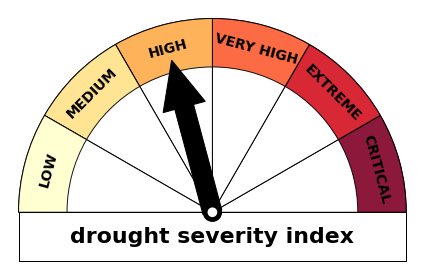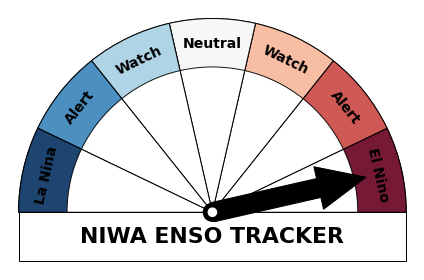# Building an ENSO tracker with Matplotlib

In this post, I will show how to play with matplotlib's patches to create a gauge or meter, the goal will be to get something like the Australian Bureau of Meteorology's ENSO tracker below#### imports¶

In :
%matplotlib inline

In :
import os, sys
import matplotlib
from matplotlib import cm
from matplotlib import pyplot as plt
import numpy as np


Looking at the BoM ENSO tracker above, it looks like we can build our tracker using a mix of circles, wedges and rectangles, we will also need an arrow, and for that we will use the arrow function of the matplotlib.pyplot.

A good place to start to have a feel of what you can do with matplotlib patches is the patch collection example code on matplotlib's website.

To dig more into each of the individual patches, head to the relevant section of the documentation on matplotlib.patches

In :
from matplotlib.patches import Circle, Wedge, Rectangle


#### some function definitions¶

below some functions to deal with some basic trigonometry that we'll be using to draw the wedges (i.e. the sectors of the gauge) and to point the arrow to the right sector

In :
def degree_range(n):
start = np.linspace(0,180,n+1, endpoint=True)[0:-1]
end = np.linspace(0,180,n+1, endpoint=True)[1::]
mid_points = start + ((end-start)/2.)
return np.c_[start, end], mid_points

In :
def rot_text(ang):
return rotation


#### main gauge function¶

In :
def gauge(labels=['LOW','MEDIUM','HIGH','VERY HIGH','EXTREME'], \
colors='jet_r', arrow=1, title='', fname=False):

"""
some sanity checks first

"""

N = len(labels)

if arrow > N:
raise Exception("\n\nThe category ({}) is greated than \
the length\nof the labels ({})".format(arrow, N))

"""
if colors is a string, we assume it's a matplotlib colormap
and we discretize in N discrete colors
"""

if isinstance(colors, str):
cmap = cm.get_cmap(colors, N)
cmap = cmap(np.arange(N))
colors = cmap[::-1,:].tolist()
if isinstance(colors, list):
if len(colors) == N:
colors = colors[::-1]
else:
raise Exception("\n\nnumber of colors {} not equal \
to number of categories{}\n".format(len(colors), N))

"""
begins the plotting
"""

fig, ax = plt.subplots()

ang_range, mid_points = degree_range(N)

labels = labels[::-1]

"""
plots the sectors and the arcs
"""
patches = []
for ang, c in zip(ang_range, colors):
# sectors
patches.append(Wedge((0.,0.), .4, *ang, facecolor='w', lw=1, edgecolor='k'))
# arcs
patches.append(Wedge((0.,0.), .4, *ang, width=0.10, facecolor=c, lw=1, edgecolor='k', alpha=0.9))

"""
set the labels (e.g. 'LOW','MEDIUM',...)
"""

for mid, lab in zip(mid_points, labels):

horizontalalignment='center', verticalalignment='center', fontsize=14, \
fontweight='bold', rotation = rot_text(mid))

"""
set the bottom banner and the title
"""
r = Rectangle((-0.4,-0.1),0.8,0.1, facecolor='w', lw=1, edgecolor='k')

ax.text(0, -0.05, title, horizontalalignment='center', \
verticalalignment='center', fontsize=22, fontweight='bold')

"""
plots the arrow now
"""

pos = mid_points[abs(arrow - N)]

"""
removes frame and ticks, and makes axis equal and tight
"""

ax.set_frame_on(False)
ax.axes.set_xticks([])
ax.axes.set_yticks([])
ax.axis('equal')
plt.tight_layout()
if fname:
fig.savefig(fname, dpi=200)


### testing¶

let's test our brand new gauge function now, using a few different colormaps

#### with a list of pre-defined colors¶

In :
gauge(labels=['LOW','MEDIUM','HIGH','EXTREME'], \
colors=['#007A00','#0063BF','#FFCC00','#ED1C24'], arrow=3, title='something here')#### using 6 instead of 5 categories, and a linear colormap¶

In :
gauge(labels=['LOW','MEDIUM','HIGH','VERY HIGH','EXTREME','CRITICAL'], \
colors='YlOrRd_r', arrow=3, title='drought severity index')#### and finally our ENSO tracker¶

In :
gauge(labels=['La Nina','Alert','Watch','Neutral','Watch','Alert','El Nino'], \
colors='RdBu', arrow=7, title='NIWA ENSO TRACKER')